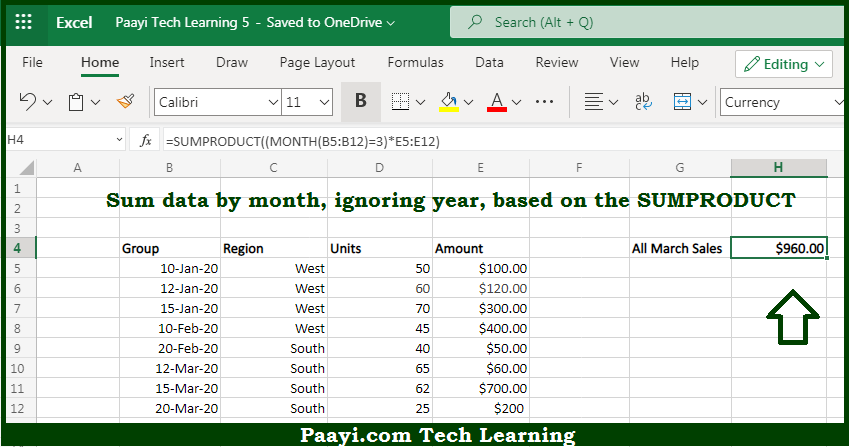# Learn How to SUM By Month Ignore Year in Microsoft Excel

Written by | 0 Comments | 671 Views

In this article, you will learn how to COUNT various things in Microsoft Excel using a single/combination(s) of functions. You will also know how to SUM By Month Ignore Year and see the generic formula.

SUM By Month Ignore Year in Microsoft Excel

The main purpose of this formula is to sum data by month, ignoring year. Here we will learn how to SUM by month and at the same time ignoring year in Microsoft Excel. That implies, with the help of a formula based on the SUMPRODUCT and MONTH function you can able to sum by month. So, with the help of this formula, you can able to SUM by month and at the same time ignoring year in Microsoft Excel.

General Formula to SUM By Month Ignore Year

=SUMPRODUCT((MONTH(dates)=month)*amounts)

The Explanation for the SUM By Month Ignore YearSo we know that with the help of the given formula above you can able to sum data by month, ignoring year. Here we will learn how to SUM by month and at the same time ignoring year in Microsoft Excel. As we know that the inside the SUMPRODUCT function, the MONTH function is used to extract the month number for every date in the data set. Besides that, it will compare it with the number provided. So, with the help of this formula, you can able to SUM by month and at the same time ignoring year in Microsoft Excel.